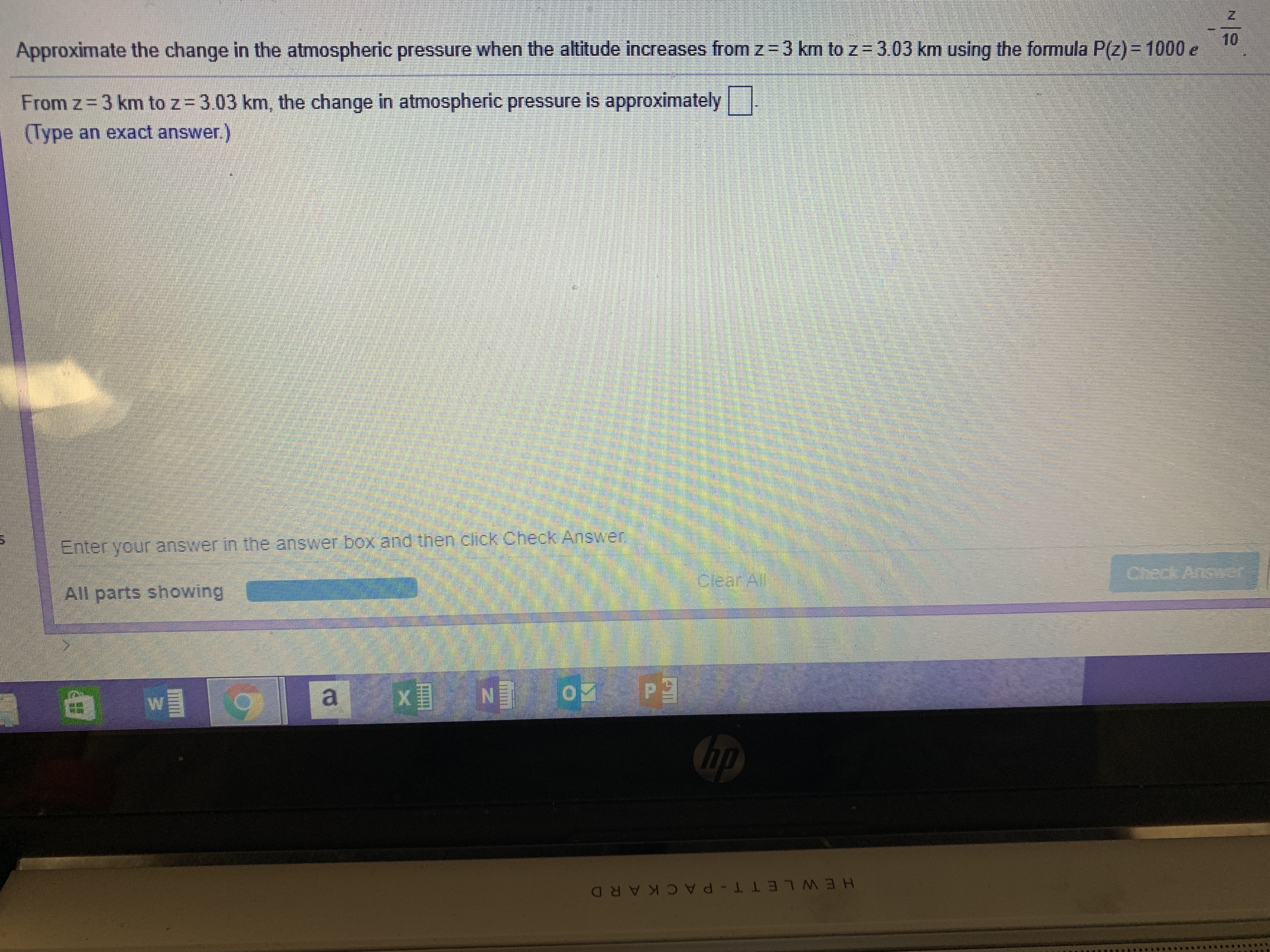# 10Approximate the change in the atmospheric pressure when the altitude increases from z = 3 km to z-303 km using the formula P z = 1000 eFrom z 3 km to z 3.03 km, the change in atmospheric pressure is approximatelyType an exact answer.)Enter your answer in the answer box and then click Check AnswerAll parts showing

Questionhelp_outlineImage Transcriptionclose10 Approximate the change in the atmospheric pressure when the altitude increases from z = 3 km to z-303 km using the formula P z = 1000 e From z 3 km to z 3.03 km, the change in atmospheric pressure is approximately Type an exact answer.) Enter your answer in the answer box and then click Check Answer All parts showing fullscreen
check_circle

Step 1

The formula for atmospheric pressure is,

Step 2

Given that, the altitude is increases from 3 to 3.03km.

When z = 3, the equation of P(z) is,

Step 3

Obtain the derivative of P(z) with respect t...

### Want to see the full answer?

See Solution

#### Want to see this answer and more?

Solutions are written by subject experts who are available 24/7. Questions are typically answered within 1 hour.*

See Solution
*Response times may vary by subject and question.
Tagged in

### Other